Maharashtra Board Class 12 Physics Solutions Chapter 3 Kinetic Theory of Gases and Radiation

Balbharti Maharashtra State Board 12th Physics Textbook Solutions Chapter 3 Kinetic Theory of Gases and Radiation Textbook Exercise Questions and Answers.

Maharashtra State Board 12th Physics Solutions Chapter 3 Kinetic Theory of Gases and Radiation

1. Choose the correct option.

i) In an ideal gas, the molecules possess
(A) only kinetic energy
(B) both kinetic energy and potential energy
(C) only potential energy
(D) neither kinetic energy nor potential energy
(A) only kinetic energy

ii) The mean free path λ of molecules is given by
(A) $$\sqrt{\frac{2}{\pi n d^{2}}}$$
(B) $$\frac{1}{\pi n d^{2}}$$
(C) $$\frac{1}{\sqrt{2} \pi n d^{2}}$$
(D) $$\frac{1}{\sqrt{2 \pi n d^{1}}}$$
where n is the number of molecules per unit volume and d is the diameter of the molecules.
(C) $$\frac{1}{\sqrt{2} \pi n d^{2}}$$iii) If pressure of an ideal gas is decreased by 10% isothermally, then its volume will
(A) decrease by 9%
(B) increase by 9%
(C) decrease by 10%
(D) increase by 11.11%
(D) increase by 11.11% [Use the formula P1V1 = P2V2. It gives $$\frac{V_{2}}{V_{1}}$$ = $$\frac{1}{0.9}$$ = 1.111 ∴ $$\frac{V_{2}-V_{1}}{V_{1}}$$ = 0.1111, i.e., 11.11%

iv) If a = 0.72 and r = 0.24, then the value of tr is
(A) 0.02
(B) 0.04
(C) 0.4
(D) 0.2
(B) 0.04

v) The ratio of emissive power of a perfect blackbody at 1327°C and 527°C is
(A) 4 : 1
(B) 16 : 1
(C) 2 : 1
(D) 8 : 1
(B) 16 : 1

i) What will happen to the mean square speed of the molecules of a gas if the temperature of the gas increases?
If the temperature of a gas increases, the mean square speed of the molecules of the gas will increase in the same proportion.
[Note: $$\overline{v^{2}}$$ = $$\frac{3 n R T}{N m}$$ ∴ $$\overline{v^{2}}$$ ∝ T for a fixed mass of gas.]

ii) On what factors do the degrees of freedom depend?
The degrees of freedom depend upon
(i) the number of atoms forming a molecule
(ii) the structure of the molecule
(iii) the temperature of the gas.

iii) Write ideal gas equation for a mass of 7 g of nitrogen gas.
In the usual notation, PV = nRT.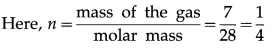Therefore, the corresponding ideal gas equation is
PV = $$\frac{1}{4}$$RT.

iv) What is an ideal gas ? Does an ideal gas exist in practice ?.
An ideal or perfect gas is a gas which obeys the gas laws (Boyle’s law, Charles’ law and Gay-Lussac’s law) at all pressures and temperatures. An ideal gas cannot be liquefied by application of pressure or lowering the temperature.

A molecule of an ideal gas is an ideal particle having only mass and velocity. Its structure and size are ignored. Also, intermolecular forces are zero except during collisions

v) Define athermanous substances and diathermanous substances.

1. A substance which is largely opaque to thermal radiations, i.e., a substance which does not transmit heat radiations incident on it, is known as an athermanous substance.
2. A substance through which heat radiations can pass is known as a diathermanous substance.

Question 3.
When a gas is heated its temperature increases. Explain this phenomenon based on kinetic theory of gases.
Molecules of a gas are in a state of continuous random motion. They possess kinetic energy. When a gas is heated, there is increase in the average kinetic energy per molecule of the gas. Hence, its temperature increases (the average kinetic energy per molecule being proportional to the absolute temperature of the gas).

Question 4.
Explain, on the basis of kinetic theory, how the pressure of gas changes if its volume is reduced at constant temperature.
The average kinetic energy per molecule of a gas is constant at constant temperature. When the volume of a gas is reduced at constant temperature, the number of collisions of gas molecules per unit time with the walls of the container increases. This increases the momentum transferred per unit time per unit area, i.e., the force exerted by the gas on the walls. Hence, the pressure of the gas increases.Question 5.
Mention the conditions under which a real gas obeys ideal gas equation.
A real gas obeys ideal gas equation when temperature is vey high and pressure is very low.
[ Note : Under these conditions, the density of a gas is very low. Hence, the molecules, on an average, are far away from each other. The intermolecular forces are then not of much consequence. ]

Question 6.
State the law of equipartition of energy and hence calculate molar specific heat of mono-and di-atomic gases at constant volume and constant pressure.
Law of equipartition of energy : For a gas in thermal equilibrium at absolute temperature T, the average energy for a molecule, associated with each quadratic term (each degree of freedom), is $$\frac{1}{2}$$kBT,
where kB is the Boltzmann constant. OR

The energy of the molecules of a gas, in thermal equilibrium at a thermodynamic temperature T and containing large number of molecules, is equally divided among their available degrees of freedom, with the energy per molecule for each degree of freedom equal to $$\frac{1}{2}$$kBT, where kB is the Boltzmann constant.
(a) Monatomic gas : For a monatomic gas, each atom has only three degrees of freedom as there can be only translational motion. Hence, the average energy per atom is $$\frac{3}{2}$$kBT. The total internal energy per mole of the gas is E = $$\frac{3}{2}$$NAkBT, where NA is the Avogadro
number.
Therefore, the molar specific heat of the gas at constant volume is
CV = $$\frac{d E}{d T}$$ = $$\frac{3}{2}$$NAkB = $$\frac{3}{2}$$R,
where R is the universal gas constant.
Now, by Mayer’s relation, Cp — Cv = R, where Cp is the specific heat of the gas at constant pressure.
∴ CP = CV + R = $$\frac{3}{2}$$R + R = $$\frac{5}{2}$$R

(b) Diatomic gas : Treating the molecules of a diatomic gas as rigid rotators, each molecule has three translational degrees of freedom and two rotational degrees of freedom. Hence, the average energy per molecule is
3($$\frac{1}{2}$$kBT) + 2($$\frac{1}{2}$$kBT) = $$\frac{5}{2}$$kBT
The total internal energy per mole of the gas is
E = $$\frac{5}{2}$$NAkBT.
∴ CV = $$\frac{d E}{d T}$$ = $$\frac{5}{2}$$NAkB = $$\frac{5}{2}$$R and
CP = CV + R = $$\frac{5}{2}$$R + R = $$\frac{7}{2}$$R
A soft or non-rigid diatomic molecule has, in addition, one frequency of vibration which contributes two quadratic terms to the energy.
Hence, the energy per molecule of a soft diatomic molecule is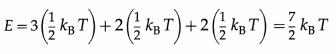Therefore, the energy per mole of a soft diatomic molecule is
E = $$\frac{7}{2}$$kBT × NA = $$\frac{7}{2}$$ RT
In this case, CV = $$\frac{d E}{d T}$$ = $$\frac{7}{2}$$R and
CP = CV + R = $$\frac{7}{2}$$R + R = $$\frac{9}{2}$$R
[Note : For a monatomic gas, adiabatic constant,
γ = $$\frac{C_{p}}{C_{V}}$$ = $$\frac{5}{3}$$. For a diatomic gas, γ =$$\frac{7}{5}$$ or $$\frac{9}{7}$$.]Question 7.
What is a perfect blackbody ? How can it be realized in practice?
A perfect blackbody or simply a blackbody is defined as a body which absorbs all the radiant energy incident on it.

Fery designed a spherical blackbody which consists of a hollow double-walled, metallic sphere provided with a tiny hole or aperture on one side, in below figure. The inside wall of the sphere is blackened with lampblack while the outside is silver-plated. The space between the two walls is evacuated to minimize heat loss by conduction and convection.

Any radiation entering the sphere through the aperture suffers multiple reflections where about 97% of it is absorbed at each incidence by the coating of lampblack. The radiation is almost completely absorbed after a number of internal reflections. A conical projection on the inside wall opposite the hole minimizes probability of incident radiation escaping out.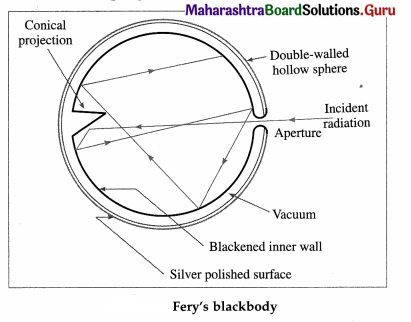When the sphere is placed in a bath of suitable fused salts, so as to maintain it at the desired temperature, the hole serves as a source of black-body radiation. The intensity and the nature of the radiation depend only on the temperature of the walls.

A blackbody, by definition, has coefficient of absorption equal to 1. Hence, its coefficient of reflection and coefficient of transmission are both zero.

The radiation from a blackbody, called blackbody radiation, covers the entire range of the electromagnetic spectrum. Hence, a blackbody is called a full radiator.

Question 8.
State (i) Stefan-Boltmann law and
(ii) Wein’s displacement law.
(i) The Stefan-Boltzmann law : The rate of emission of radiant energy per unit area or the power radiated per unit area of a perfect blackbody is directly proportional to the fourth power of its absolute temperature. OR
The quantity of radiant energy emitted by a perfect blackbody per unit time per unit surface area of the body is directly proportional to the fourth power of its absolute temperature.

(ii) Wien’s displacement law : The wavelength for which the emissive power of a blackbody is maximum, is inversely proportional to the absolute temperature of the blackbody.
OR
For a blackbody at an absolute temperature T, the product of T and the wavelength λm corresponding to the maximum radiation of energy is a constant.
λmT = b, a constant.
[Notes: (1) The law stated above was stated by Wilhelm Wien (1864-1928) German Physicist. (2) The value of the constant b in Wien’s displacement law is 2.898 × 10-3 m.K.]

Question 9.
Explain spectral distribution of blackbody radiation.
Blackbody radiation is the electromagnetic radiation emitted by a blackbody by virtue of its temperature. It extends over the whole range of wavelengths of electromagnetic waves. The distribution of energy over this entire range as a function of wavelength or frequency is known as the spectral distribution of blackbody radiation or blackbody radiation spectrum.

If Rλ is the emissive power of a blackbody in the wavelength range λ and λ + dλ, the energy it emits per unit area per unit time in this wavelength range depends on its absolute temperature T, the wavelength λ and the size of the interval dλ.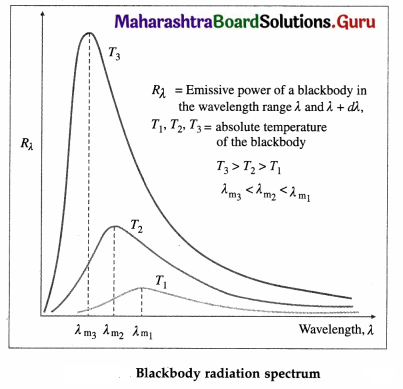Question 10.
State and prove Kirchoff’s law of heat radiation.
Kirchhoff’s law of heat radiation : At a given temperature, the ratio of the emissive power to the coefficient of absorption of a body is equal to the emissive power of a perfect blackbody at the same temperature for all wavelengths.
OR
For a body emitting and absorbing thermal radiation in thermal equilibrium, the emissivity is equal to its absorptivity.

Theoretical proof: Consider the following thought experiment: An ordinary body A and a perfect black body B are enclosed in an athermanous enclosure as shown in below figure.

According to Prevost’s theory of heat exchanges, there will be a continuous exchange of radiant energy between each body and its surroundings. Hence, the two bodies, after some time, will attain the same temperature as that of the enclosure.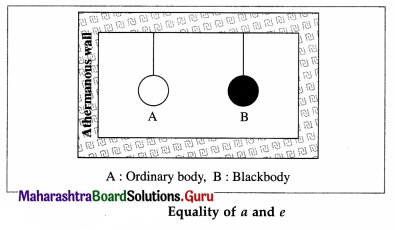Let a and e be the coefficients of absorption and emission respectively, of body A. Let R and Rb be the emissive powers of bodies A and B, respectively. ;

Suppose that Q is the quantity of radiant energy incident on each body per unit time per unit surface area of the body.

Body A will absorb the quantity aQ per unit time per unit surface area and radiate the quantity R per unit time per unit surface area. Since there is no change in its temperature, we must have,
aQ = R … (1)
As body B is a perfect blackbody, it will absorb the quantity Q per unit time per unit surface area and radiate the quantity Rb per unit time per unit surface area.

Since there is no change in its temperature, we must have,
Q = Rb ….. (2)
From Eqs. (1) and (2), we get,
a = $$\frac{R}{Q}$$ = $$\frac{R}{R_{\mathrm{b}}}$$ ….. (3)
From Eq. (3), we get, $$\frac{R}{a}$$ = Rb OR

By definition of coefficient of emission,
$$\frac{R}{R_{\mathrm{b}}}$$ …(4)
From Eqs. (3) and (4), we get, a = e.
Hence, the proof of Kirchhoff ‘s law of radiation.Question 11.
Calculate the ratio of mean square speeds of molecules of a gas at 30 K and 120 K. [Ans: 1:4]
Data : T1 = 30 K, T2 = 120 K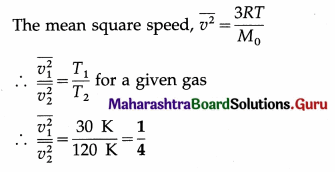This is the required ratio.

Question 12.
Two vessels A and B are filled with same gas where volume, temperature and pressure in vessel A is twice the volume, temperature and pressure in vessel B. Calculate the ratio of number of molecules of gas in vessel A to that in vessel B.
[Ans: 2:1]
Data : VA = 2VB, TA = 2TB, PA = 2PB PV = NkBT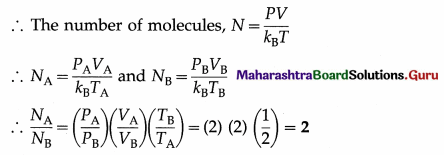This is the required ratio.

Question 13.
A gas in a cylinder is at pressure P. If the masses of all the molecules are made one third of their original value and their speeds are doubled, then find the resultant pressure. [Ans: 4/3 P]
Data : m2 = m1/3, vrms 2 = 2vrms 1 as the speeds of all molecules are doubled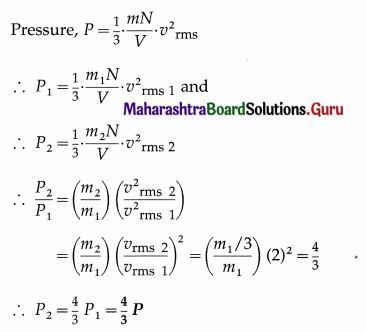This is the resultant pressure.

Question 14.
Show that rms velocity of an oxygen molecule is $$\sqrt{2}$$ times that of a sulfur dioxide molecule at S.T.P.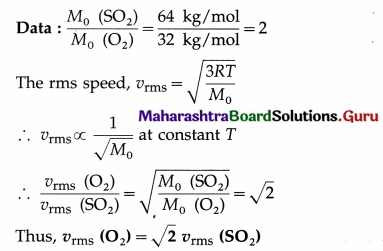Question 15.
At what temperature will oxygen molecules have same rms speed as helium molecules at S.T.P.? (Molecular masses of oxygen and helium are 32 and 4 respectively)
[Ans: 2184 K]
Data : T2 = 273 K, M01 (oxygen) = 32 × 10-3 kg/mol, M02 (hydrogen) = 4 × 10– 3 kg/mol
The rms speed of oxygen molecules, v1 = $$\sqrt{\frac{3 R T_{1}}{M_{01}}}$$ and that of helium molecules, v2 = $$\sqrt{\frac{3 R T_{2}}{M_{02}}}$$
When v1 = v2,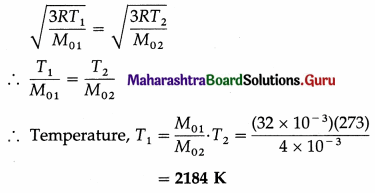Question 16.
Compare the rms speed of hydrogen molecules at 127 ºC with rms speed of oxygen molecules at 27 ºC given that molecular masses of hydrogen and oxygen are 2 and 32 respectively. [Ans: 8: 3]
Data : M01 (hydrogen) = 2 g/mol,
M02 (oxygen) = 32 g/mol,
T1 (hydrogen) = 273 + 127 = 400 K,
T2 (oxygen) = 273 + 27 = 300 K
The rms speed, vrms = $$\sqrt{\frac{3 R T}{M_{0}}}$$,
where M0 denotes the molar mass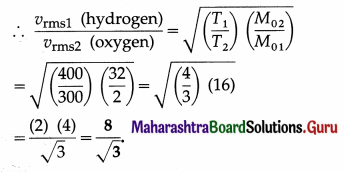Question 17.
Find kinetic energy of 5000 cc of a gas at S.T.P. given standard pressure is 1.013 × 105 N/m2.
[Ans: 7.598 × 102 J]
Data : P = 1.013 × 105 N/m2, V = 5 litres
= 5 × 10-3 m3
E = $$\frac{3}{2}$$PV
= $$\frac{3}{2}$$(1.013 × 105 N/m2) (5 × 10-3 m3)
= 7.5 × 1.013 × 102 J = 7.597 × 102 J
This is the required energy.Question 18.
Calculate the average molecular kinetic energy
(i) per kmol
(ii) per kg
(iii) per molecule of oxygen at 127 ºC, given that molecular weight of oxygen is 32, R is 8.31 J mol-1 K-1 and Avogadro’s number NA is 6.02 × 1023 molecules mol-1.
[Ans: 4.986 × 106J, 1.558 × 102J 8.282 × 10-21 J]
Data : T = 273 +127 = 400 K, molecular weight = 32 ∴ molar mass = 32 kg/kmol, R = 8.31 Jmol-1 K-1, NA = 6.02 × 1023 molecules mol-1

(i) The average molecular kinetic energy per kmol of oxygen = the average kinetic energy per mol of oxygen × 1000
= $$\frac{3}{2}$$RT × 1000 = $$\frac{3}{2}$$ (8.31) (400) (103)$$\frac{\mathrm{J}}{\mathrm{kmol}}$$
= (600)(8.31)(103) = 4.986 × 106 J/kmol

(ii) The average molecular kinetic energy per kg of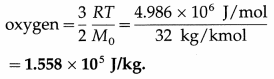(iii) The average molecular kinetic energy per molecule of oxygen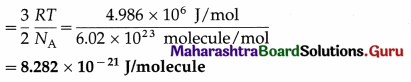Question 19.
Calculate the energy radiated in one minute by a blackbody of surface area 100 cm2 when it is maintained at 227ºC. (Take Stefen’s constant σ = 5.67 × 10-8J m-2s-1K-4)
[Ans: 2126.25 J]
Data : t = one minute = 60 s, A = 100 cm2
= 100 × 10-4 m2 = 10-2 m2, T = 273 + 227 = 500 K,
σ = 5.67 × 10-8 W/m2.K4
The energy radiated, Q = σAT4t
= (5.67 × 10-8)(10-2)(500)4(60) J
= (5.67)(625)(60)(10-2)J = 2126 J

Question 20.
Energy is emitted from a hole in an electric furnace at the rate of 20 W, when the temperature of the furnace is 727 ºC. What is the area of the hole? (Take Stefan’s constant σ to be 5.7 × 10-8 J s-1 m-2 K-4) [Ans: 3.509 × 10-4m2]
Data : $$\frac{Q}{t}$$ = 20 W, T = 273 + 727 = 1000 K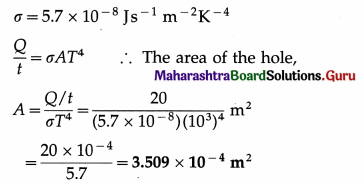Question 21.
The emissive power of a sphere of area 0.02 m2 is 0.5 kcal s-1 m-2. What is the
amount of heat radiated by the spherical surface in 20 second? [Ans: 0.2 kcal]
Data : R = 0.5 kcal s-1m-2, A = 0.02 m2, t = 20 s Q = RAt = (0.5) (0.02) (20) = 0.2 kcal
This is the required quantity.

Question 22.
Compare the rates of emission of heat by a blackbody maintained at 727ºC and at 227ºC, if the black bodies are surrounded
by an enclosure (black) at 27ºC. What would be the ratio of their rates of loss of heat ?
[Ans: 18.23:1]
Data : T1 = 273 + 727 = 1000 K, T2 = 273 + 227 = 500 K, T0 = 273 + 27 = 300 K.
(i) The rate of emission of heat, $$\frac{d Q}{d t}$$ = σAT4.
We assume that the surface area A is the same for the two bodies.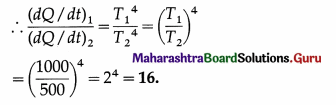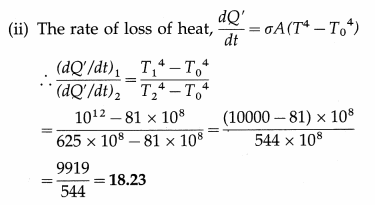Question 23.
Earth’s mean temperature can be assumed to be 280 K. How will the curve of blackbody radiation look like for this temperature? Find out λmax. In which part of the electromagnetic spectrum, does this value lie? (Take Wien’s constant b = 2.897 × 10-3 m K) [Ans: 1.035 × 10-5m, infrared region]
Data : T = 280 K, Wien’s constant b = 2.897 × 10-3 m.K
λmaxT = b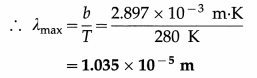This value lies in the infrared region of the electromagnetic spectrum.
The nature of the curve of blackbody radiation will be the same as shown in above, but the maximum will occur at 1.035 × 10-5 m.

Question 24.
A small-blackened solid copper sphere of radius 2.5 cm is placed in an evacuated chamber. The temperature of the chamber is maintained at 100 ºC. At what rate energy must be supplied to the copper sphere to maintain its temperature at 110 ºC? (Take Stefan’s constant σ to be 5.670 × 10-8 J s-1 m-2 K-4 , π = 3.1416 and treat the sphere as a blackbody.)
[Ans: 0.9624 W]
Data : r = 2.5 cm = 2.5 × 10-2 m, T0 = 273 + 100 = 373 K, T = 273 + 110 = 383 K,
σ = 5.67 × 10-8 J s-1 m-2 k-4
The rate at which energy must be supplied
σA(T4 — T04) = σ 4πr2(T4 – T04)
= (5.67 × 10-8) (4) (3.142) (2.5 × 10-2)2 (3834 – 3734)
= (5.67) (4) (3.142) (6.25) (3.834 – 3.734) × 10-4
= 0.9624WQuestion 25.
Find the temperature of a blackbody if its spectrum has a peak at (a) λmax = 700 nm (visible), (b) λmax = 3 cm (microwave region) and (c) λmax = 3 m (short radio waves) (Take Wien’s constant b = 2.897 × 10-3 m K). [Ans: (a) 4138 K, (b) 0.09657 K, (c) 0.9657 × 10-3 K]
Data:(a) λmax = 700nm=700 × 10-9m,
(b) λmax = 3cm = 3 × 10-2 m, (c) λmax = 3 m, b = 2.897 × 10-3 m.K
λmaxT = b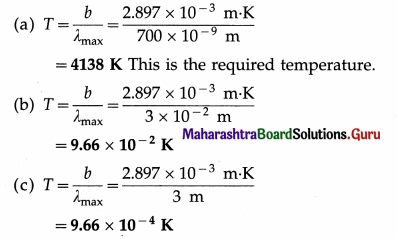12th Physics Digest Chapter 3 Kinetic Theory of Gases and Radiation Intext Questions and Answers

Remember This (Textbook Page No. 60)

Question 1.
Distribution of speeds of molecules of a gas.
Maxwell-Boltzmann distribution of molecular speeds is a relation that describes the distribution of speeds among the molecules of a gas at a given temperature.

The root-mean-square speed vrms gives us a general idea of molecular speeds in a gas at a given temperature. However, not all molecules have the same speed. At any instant, some molecules move slowly and some very rapidly. In classical physics, molecular speeds may be considered to cover the range from 0 to ∞. The molecules constantly collide with each other and with the walls of the container and their speeds change on collisions. Also the number of molecules under consideration is very large statistically. Hence, there is an equilibrium distribution of speeds.

If dNv represents the number of molecules with speeds between v and v + dv, dNv remains fairly constant at equilibrium. We consider a gas of total N molecules. Let r\vdv be the probability that a molecule has its speed between v and v + dv. Then, dNv = Nηvdv
so that the fraction, i.e., the relative number of molecules with speeds between v and v + dv is dNv/N = ηvdv
Below figure shows the graph of ηv against v. The area of the strip with height ηv and width dv gives the fraction dNv/N.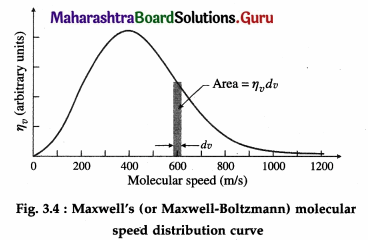Remember This (Textbook Page No. 64)

Question 1.
If a hot body and a cold body are kept in vacuum, separated from each other, can they exchange heat ? If yes, which mode of transfer of heat causes change in their temperatures ? If not, give reasons.

Remember This (Textbook Page No. 66)

Question 1.
Can a perfect blackbody be realized in practice ?
For almost all practical purposes, Fery’s blackbody is very close to a perfect blackbody.

Question 2.
Are good absorbers also good emitters ?
Yes.Use your brain power (Textbook Page No. 68)

Question 1.
Why are the bottom of cooking utensils blackened and tops polished ?
The bottoms of cooking utensils are blackened to increase the rate of absorption of radiant energy and tops are polished to increase the reflection of radiation.

Question 2.
A car is left in sunlight with all its windows closed on a hot day. After some time it is observed that the inside of the car is warmer than outside air. Why ?